15min

# Getting Started with Python - Part 2

Part 1 of article series can be found here.

### FUNCTION DEFINITION AND CALL

Functions provide a systematic way to solve a problem with a methodical approach. By dividing the solution into sub-problems and solving each one at a time, this makes the problem solving more modular. Python insists on strict indentation rules.

#### DEFINING A FUNCTION:

Function is defined by using a keyword def, that should not be the same as any built-in function name. The statement inside the function should be indented with respect to the left margin. It makes the code look more elegant and it is also a requirement of Python that the code following the colon be indented.

``````1
2
``````def function_name(parameters_separated_by_comma):
statements
``````

#### CALLING A FUNCTION:

To call the function we need to name the function followed by parentheses () after the function is defined. If any parameter is required we need to give that inside the parentheses.

Example: Creating a function to print a triangle and square.

``````1
2
3
4
5
6
7
8
9
10
11
12
13
14
15
``````def diagram():
print("   *")
print("  * *")
print(" * * *")
print("* * * *")

print() # Print a new blank line

print("* " * 4) # Print * 4 times
print("* " * 4)
print("* " * 4)
print("* " * 4)

diagram() # Calling the diagram
function.
``````

Functions can also be called inside another function.

Example: This will give the same output as earlier.

``````1
2
3
4
5
``````def triangle():
print("   *")
print("  * *")
print(" * * *")
print("* * * *")
``````
``````1
2
``````def newLine():
print() # Print a new blank line
``````
``````1
2
3
4
5
``````def square():
print("* " * 4) # Print * 4 times
print("* " * 4)
print("* " * 4)
print("* " * 4)
``````
``````1
2
3
4
5
6
7
8
9
10
``````def all():
triangle() # Calling the triangle
function.
newLine() # Calling the newLine
function.
square() # Calling the square
function.

all() # Finally calling the all
function which calls the other functions.
``````

#### FUNCTIONS CAN BE CALLED IN DIFFERENT WAYS

``````1
2
3
4
5
6
7
8
9
10
11
12
13
14
``````def add(number1, number2):
s = number1 + number2
print(s) # Printing the value inside the
function

def multiply(number1, number2):
m = number1 * number2
return m

result = multiply(4, 5) # Returning the value and printing the value outside the
function
print(result) # By storing inside Different variable.
``````

Try it yourself:

1. Create a function named volume() which takes three values, length, width and height and returns the volume.

2. Create a function named operation() which takes two numbers and prints their sum, difference, quotient, remainder and product inside the function.

### IMPORTING USER DEFINED MODULES:

Python has a huge library of functions which can be imported as per a user’s usage. For example, if we need floor, ceil, square root, etc. we need to import math library. To ensure the library is accessible to the program, it needs to be imported, which can be done using the following syntax:

`import name-of-the-module`

``````1
2
3
4
5
6
7
8
9
10
11
12
13
``````import math
from datetime
import datetime

def imports():
number = int(input("Enter the number: "))
squareRoot = math.sqrt(number)
print(squareRoot)

now = datetime.now()
print("Current time is", now)

imports()
``````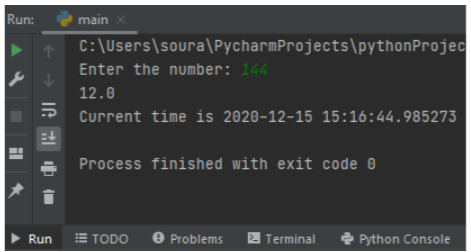### USING COMMAND LINE TO RUN FILES

Open command prompt / terminal and move to the folder where the file is saved. Type:

`python main.py or py main.py`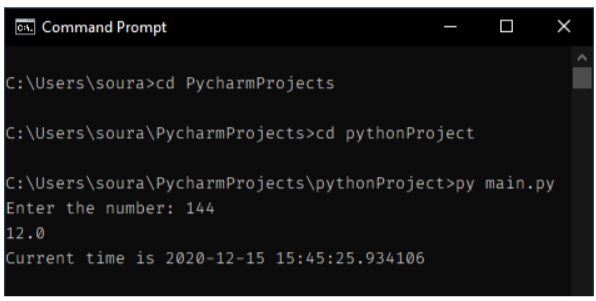TRY IT YOURSELF:

1. Write a function area that takes the length of three sides: s1, s2, and s3 of the triangle as the input parameters and returns the area of the triangle as the output.

2. Consider the following function:

``````1
2
3
4
5
6
7
8
9
10
11
``````def nMultiple(a = 0, num = 1):
return a * num

# What w ill be the output produced when the following

1.nMultiple(5)
2.nMultiple(5, 6)
3.nMultiple(num = 7)
4.nMultiple(num = 6, a = 5)
5.nMultiple(5, num = 6)
``````

### CONDITIONAL STRUCTURES

#### IF / ELSE / ELIF Conditional statement:

If and else conditional statements are used when we are required to make decisions, if a particular condition is satisfied then execute this, or else this. if statements can be used independently. Elif is used when we need to check more than two conditions. The syntax of and if-else conditional statements is as follows:

``````1
2
3
4
5
6
7
8
9
``````if <condition >:
<
Sequence of statements to be executed >
elif:
<
Sequence of statements to be executed >
else :
<
Sequence of statements to be executed >
``````

``````1
2
3
4
5
6
7
8
9
10
11
12
13
14
15
16
17
``````pwd = "mypassword@123"
previouspwd = "myPrevious"

def enter():

enter()

else :
enter()
enter()
``````#### NESTED CONDITIONAL STATEMENTS:

Conditional statements inside another conditional statement are called nested conditional statements. These can be very useful in performing complex operations.

``````1
2
3
4
5
6
7
8
9
10
11
12
13
14
``````if <condition1 >:
if < condition2 >:
<
Sequence of statements to be executed >
else :
<
Sequence of statements to be executed >
else :
if < condition3 >:
<
Sequence of statements to be executed >
else :
<
Sequence of statements to be executed >
``````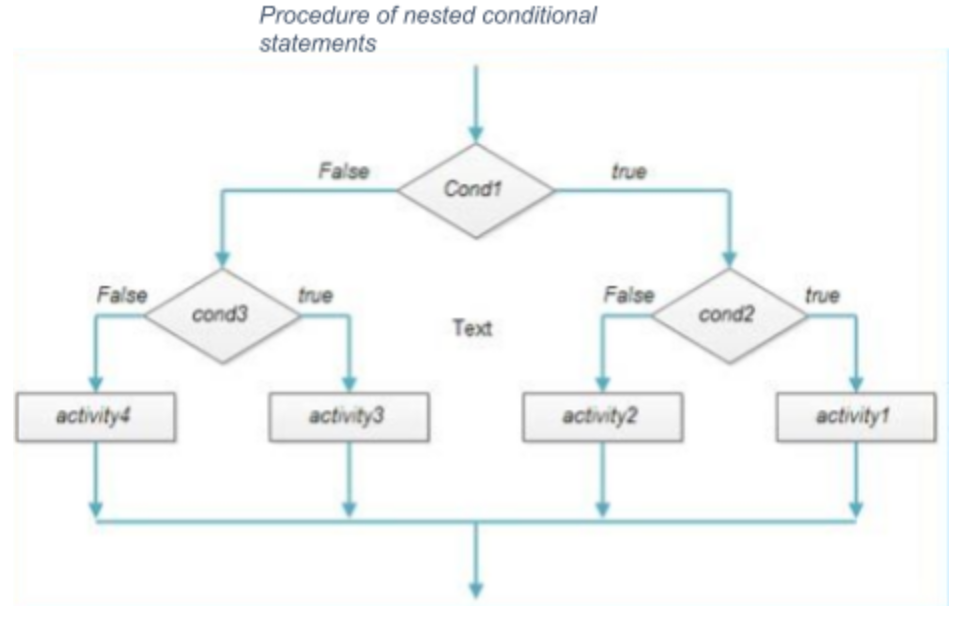EXAMPLE: Input three integers and print the maximum of them, without using `max()` function or any logical operator.

``````1
2
3
4
5
6
7
8
9
10
11
12
13
14
``````a = int(input("Enter the first No.  "))
b = int(input("Enter the second No. "))
c = int(input("Enter the third No.  "))

if a > b:
if a > c:
print(a, " is the biggest")
else :
print(c, "is the greatest")
else :
if b > c:
print(b, " is the biggest")
else :
print(c, "is the biggest")
``````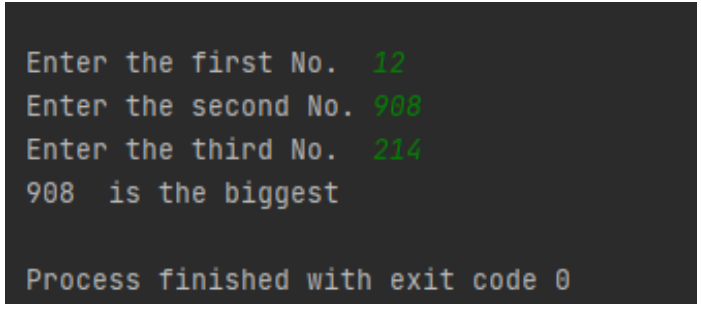TRY IT YOURSELF:

1. Create a Python program to input the marks in five subjects, full marks 100, calculate the percentage and give the remark according to percentage obtained:
100 – 80: Excellent, 80-60: good, 60-40: Work Hard, 40-0: Fail need retest

2. Create a Python program to input three integers and print the minimum of it, without using `min()` function or any logical operator.

### ITERATIONS (FOR AND WHILE STATEMENT):

The process of repetitive execution of a single statement or a sequence of statements is called a loop. Using loops, we need to write those statements only once. Execution of this sequence in a loop is known as iteration of loop.

#### FOR LOOP:

This control statement is used when we want to execute a series of statements a fixed number of times. Suppose we want to find the sum of first n positive integers. So, for that we need a counting mechanism to count from 1 to n, and we keep adding it into the current value of the old value. To achieve this, we use loops. For loops exclude the ending as the loop starts from 0. To deep dive into for loops click here.

The general form of for structure is as follows:

``````1
2
3
``````for variable in range(start, end, increment):
<
block of statements to be executed >
``````

Example 1: Take input n and print the first numbers and finally print their sum using for loop.

``````1
2
3
4
5
6
7
8
``````n = int(input("Enter the value of n -> "))
sum_value = 0 # Initialising the sum value to 0

for i in range(1, n + 1): # n + 1 as the ending value is excluded.
print(i) # Printing the number
sum_value = sum_value + i # Storing the value in sum_value.

print("Sum is", sum_value)
``````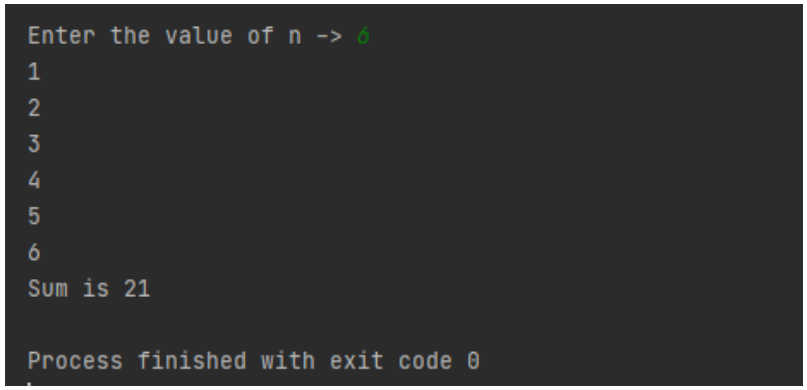Example 2: Input an integer n and check whether it is prime or not using for loop.

``````1
2
3
4
5
6
7
8
9
10
11
12
13
14
``````n = int(input("Enter the test number :"))

for i in range(2, n): # To check whether it is divisible by any number between 2 to n - 1;
if n % i == 0:
print("Number is not prime. ")
break #
break helps to
break out of the loop
# without moving the loop more further

# at last
if no number between 2 to n - 1 didn 't divide number.
if i == n - 1: # as the iterator increases to n - 1 terms only.
print("Number is prime.")
``````

#### BREAK AND CONTINUE:

Break allows us to terminate the loop as a particular condition is satisfied. After a break statement is executed, the loop doesn’t iterate further.

``````1
2
3
4
5
``````	if <condition >:
break
Continue allows us to skip a particular condition and do nothing, but it doesn’ t terminate the loop.
if < condition >:
continue
``````

#### WHILE LOOP:

With the while loop we can execute a set of statements as long as a condition is true.

``````1
2
``````	while < set_of_conditions >:
<set_of_statements_to_be_executed>
``````

EXAMPLE 1: Take input n and print the first numbers and finally print their sum using while loop.

``````1
2
3
4
5
6
7
8
9
10
``````n = int(input("Enter the limit  :"))
i = 1 # initialising the iterator
sum_value = 0
while i <= n: # conditions
print(i)
sum_value = sum_value + i

i = i + 1 # incremented

print("sum is : ", sum_value) # printing
``````

Example 2: Input an integer n and check whether it is prime or not using while loop.

n = int(input(“Enter the test number :”))
i = 2
while i < n:
if n % i == 0:
print("Number is not prime. ")
break

```i = i + 1 # incrementer ```

if i == n - 1:
print(“Number is prime.”)

This will give the same output as in the case of for loop.

TRY IT YOURSELF USING BOTH FOR AND WHILE LOOP:

1. Input an integer n and print the even numbers and odd numbers from 1 to n.

2. Print the first twenty multiples of four.

### NESTED LOOPS

Loops used inside another loop are termed nested loops. They can be performed both in for and while loop. Note: The variables inside the nested loops can’t be the same.

``````1
2
3
4
5
6
7
8
``````for variable in range(start, end, step):
for variable in range(start, end, step):
while < set_of_condition >:
<statements_to_be_executed>
increment.
while <set_of_condition>:
<statements_to_be_executed>
increment.
``````

Examples: Printing triangles with stars?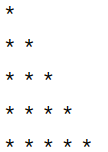Steps:

1. Every line contains the same number of stars as iteration of the loop, i.e. value of i.

2. After printing each character, the cursor needs to remain in the same line, hence end function is used.

3. After printing each line we need to move a new line, hence we used an empty `print()` function.

4. The first loop is used to iterate over the rows and the second loop is used to print the number of stars which is equal to the variable in the second loop.

``````1
2
3
4
5
6
``````n = int(input("Enter the number of lines :"))

for i in range(0, n + 1):
for j in range(0, i):
print("*", end = ' ')
print()
``````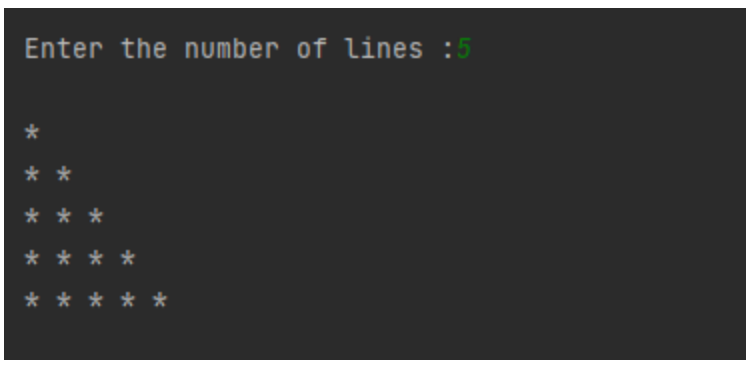Example 2: Print the prime numbers between two limits, upper and lower, given by user

``````1
2
3
4
5
6
7
8
9
10
11
12
13
``````# Take the input from the user:
lower = int(input("Enter lower range: "))
upper = int(input("Enter upper range: "))

print("Prime numbers from ", lower, " to ", upper, "is :")

for num in range(lower, upper + 1): #number is given to the internal loop:
if num > 1:
for i in range(2, num):
if (num % i) == 0:
break
else :
print(num, end = " ")
``````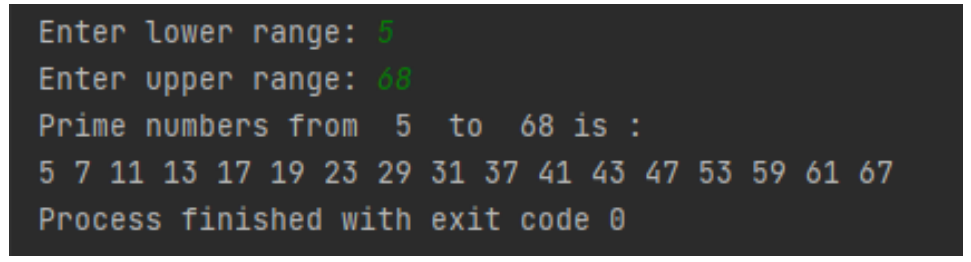Example 3: Print the following 2D figure.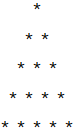STEPS:

1. There are spaces before every star which is the same as (n-I ) times every loop.

2. Every line contains the same number of stars as iteration of the loop, i.e. value of i.

3. After printing each character, the cursor needs to remain in the same line, hence end function is used.

4. After printing each line we need to move a new line, hence we used an empty `print()` function.

5. The first loop is used to iterate over the rows and the second loop is used to print the number of stars which is equal to the variable in the second loop.

``````1
2
3
4
5
6
``````for i in range(0, n + 1):
for k in range(0, n - i):
print(" ", end = '')
for j in range(0, i):
print("*", end = ' ')
print()
``````

TRY IT YOURSELF:

1. Write a program to find the factorial number.

2. Write a program to print the following 2D figure: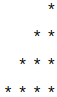### Getting Started with Python

This article will cover almost everything you need to get started with Python. Python has become one of the mo...